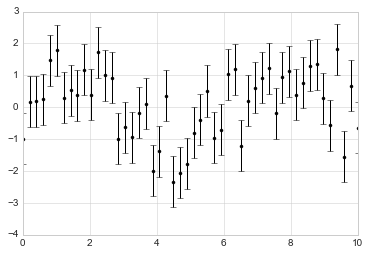# 8.6 可视化误差

## 基本的误差栏

``````%matplotlib inline
import matplotlib.pyplot as plt
plt.style.use('seaborn-whitegrid')
import numpy as np

x = np.linspace(0, 10, 50)
dy = 0.8
y = np.sin(x) + dy * np.random.randn(50)

plt.errorbar(x, y, yerr=dy, fmt='.k');
````````````plt.errorbar(x, y, yerr=dy, fmt='o', color='black',
ecolor='lightgray', elinewidth=3, capsize=0);
``````## 连续误差

``````from sklearn.gaussian_process import GaussianProcess

# 定义模型并绘制一些数据
model = lambda x: x * np.sin(x)
xdata = np.array([1, 3, 5, 6, 8])
ydata = model(xdata)

# 拟合高斯过程
gp = GaussianProcess(corr='cubic', theta0=1e-2, thetaL=1e-4, thetaU=1E-1,
random_start=100)
gp.fit(xdata[:, np.newaxis], ydata)

xfit = np.linspace(0, 10, 1000)
yfit, MSE = gp.predict(xfit[:, np.newaxis], eval_MSE=True)
dyfit = 2 * np.sqrt(MSE)  # 2*sigma ~ 95% confidence region
``````

``````# 可视化结果
plt.plot(xdata, ydata, 'or')
plt.plot(xfit, yfit, '-', color='gray')

plt.fill_between(xfit, yfit - dyfit, yfit + dyfit,
color='gray', alpha=0.2)
plt.xlim(0, 10);
``````